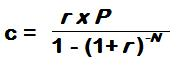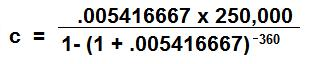CalcuNation

# Monthly Payment Mortgage Calculator

## Use this Mortgage Calculator to calculate the monthly mortgage payment on a fixed-rate mortgage.

Principal Loan Amount:

Yearly Interest Rate: %

Total Months of the Loan:

A 30 year loan has 360 months, a 15 year loan has 180 months.

### What is the mortgage payment?

To calculate the monthly payment on a mortgage loan use the formula:c = Monthly Payment

r = Monthly Interest Rate (in Decimal Form) =
(Yearly Interest Rate/100) / 12

P = Principal Amount on the Loan

N = Total # of Months for the loan ( Years on the loan x 12)

Example: Monthly mortgage payment for 30 year fixed-rate loan,
with a principal of \$250,000, and a yearly interest rate of 6.5%:

r = (6.5 / 100) / 12 = .005416667

P = 250,000

N = (30 x 12) = 360The Monthly Mortgage Payment is \$1580.17

### \$100 Promotion

Win \$100 towards teaching supplies! We want to see your websites and blogs.Enter Here

### Calculator Popups

Scientific Calculator
Simple Calculator

### Calculator Ideas

We use your calculator ideas to create new and useful online calculators.
Submit Calculator Idea